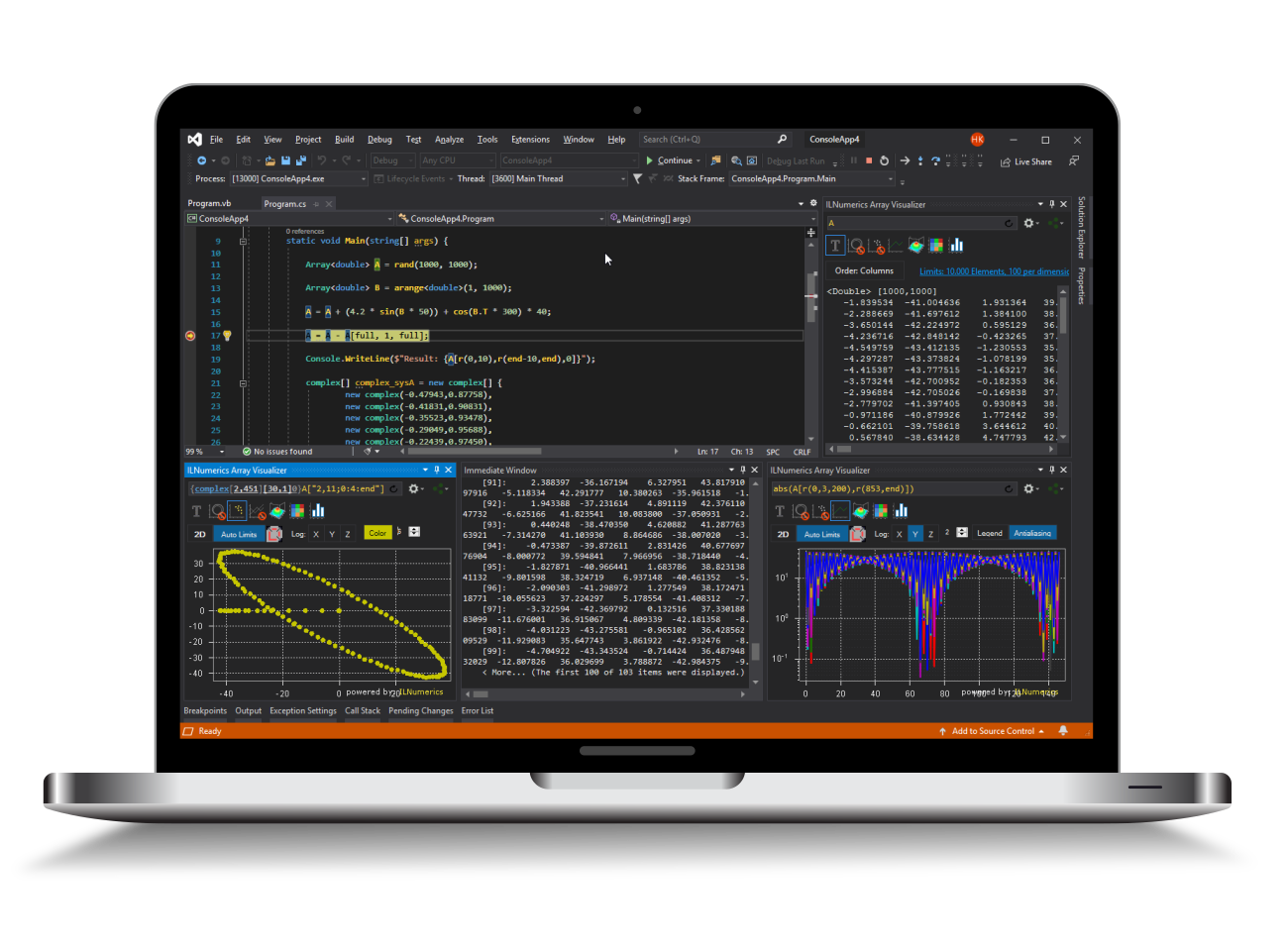Industrial Data Science
in C# and .NET:
Simple. Fast. Reliable.ILNumerics - Technical Computing

Modern High Performance Tools for Technical

Computing and Visualization in Industry and Science

tgt

# Eigenvalues and -vectors

### Symmetric Eigenproblems

Eigenvalues λi of a square or hermitian matrix A are those scalar values for which vectors vi≠0 exist such that

Av = λv.

In both cases the Eigenvalues λ are real. Considering the sets of all Eigenvalues λ1,...,λk and Eigenvectors v1,...,vk of a matrix A we may write

A = V Λ VT,

where Λ is the diagonal matrix whose diagonal elements are the Eigenvalues and V is the orthogonal (or unitary) matrix whose columns are the corresponding Eigenvectors.

### Non-Symmetric Eigenproblems

The case of non-symmetric matrices A is largely identical to the symmetric case, except that the Eigenvalues λ may be complex. Moreover, in the symmetric case there is no difference between right Eigenvectors (see above) and left Eigenvecors given by

vTA = λvT.

### Non square matrices

Eigenvectors and Eigenvalues are only defined for square matrices. For general m x n matrices a related concept exists, the so called singular value decomposition (SVD). For more details please check the next section or the class reference for ILMath.svd.

### Generalizes Eigenproblems

Another extension of Eigenproblems are the so called generalized Eigenvalues and Eigenvectors. These are given by the solutions to

Av = λBv,

where A and B are symmetric (or hermitian) matrices and B is positive definite. This again may be generalized to the non-symmetric case or general m x n matrices (i.e. generalized SVD).